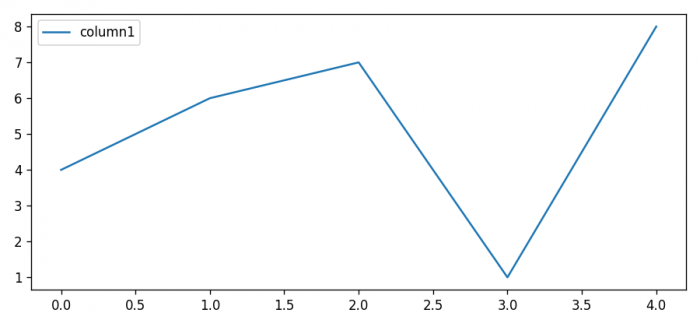# How to change the DPI of a Pandas Dataframe Plot in Matplotlib?

To change the DPI of a Pandas DataFrame plot, we can use rcParams to set the dot per inch.

## Steps

• Set the figure size and adjust the padding between and around the subplots.
• Set the DPI values in .rcParams["figure.dpi"] = 120
• Create a Pandas dataframe to make a plot.
• Plot the dataframe.
• To display the figure, use show() method.

## Example

import pandas as pd
from matplotlib import pyplot as plt

plt.rcParams["figure.figsize"] = [7.50, 3.50]
plt.rcParams["figure.autolayout"] = True

plt.rcParams["figure.dpi"] = 120

data = pd.DataFrame({"column1": [4, 6, 7, 1, 8]})
data.plot()

plt.show()

## Output